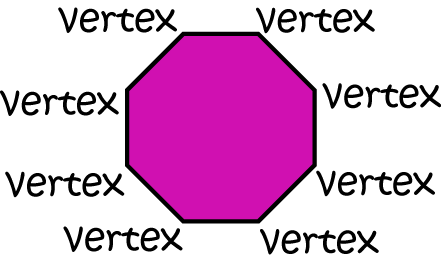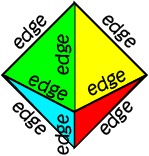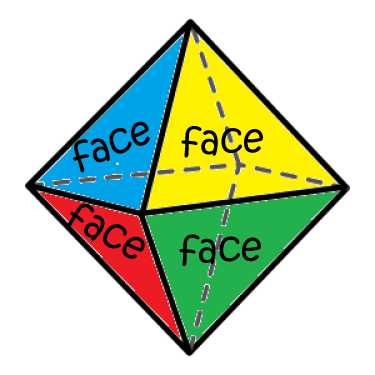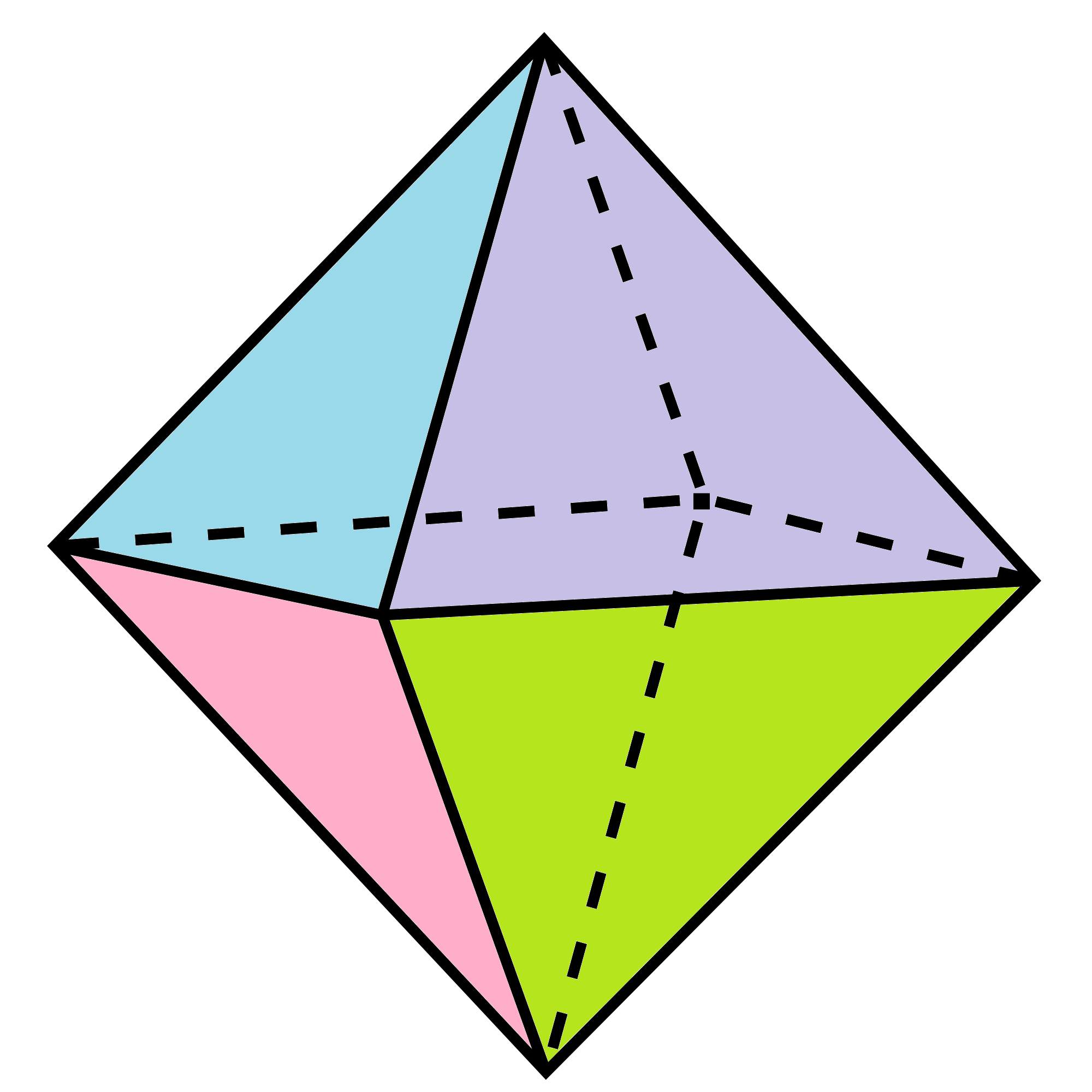# Vertices, Edges and Faces

Do you know what polyhedrons and polygons have in common? Well, there are plenty of things, but most importantly for this article, both have vertices (the plural of vertex) and edges. A polygon can be a face of a polyhedron, so polyhedra (the plural of polyhedron) have vertices, faces and edges. Let's see what each of these three terms means.

Informally speaking, a  vertex  is a corner, an edge is a straight line that joins two corners, and a face is a flat surface on a polyhedron. Let's try and come up with some definitions of these things that will make those fuss-pots, the mathematicians, happier.## VerticesVertices are the pointy bits that form the corners of polyhedra and polygons. I don't think that is going to cut it with the mathematicians, do you? How about: "a vertex is a point where two or more lines intersect". That sounds a bit more formal. Between ourselves, I think of vertices as corners, but it pays to keep the mathematicians happy sometimes.

The square pyramid in the picture on the left has 5 vertices, while the octagon in the picture on the right has 8.## EdgesHow about an edge? Well, remember that a straight line is infinite, so we'd better call an edge a line segment. How does: "An edge is a line segment that joins two vertices of a polygon" sound? That's pretty good, I think, but what about an edge on a polyhedron? This should do the trick: "an edge is a line segment at which two faces intersect." Sounds good to me! Sometimes, edges of polygons are also known as sides, but then, just to make things more confusing, faces of polyhedra are often called sides.

The octahedron in the picture on the left has $12$ edges. The pentagon in the picture on the right has $5$.## FacesAnd finally, we need to define faces. Well, there's not much more that we can say: a face is one of the individual flat surfaces of a solid object. Polyhedrons are bounded entirely by faces. This octahedron has eight faces (there are four you cannot see).

## Euler's Formula

Mathematicians have been fascinated by the properties of polyhedra since the time of the Ancient Greeks, and probably even earlier. The Swiss mathematician, Leonhard Euler (1707-1783) appears to have been the first to notice that, for convex polyhedra (polyhedra without any dents or gaps), there is a simple relationship between the numbers of vertices, edges and faces. This is summarised in Euler's Formula, which states that

$F + V- E = 2$,
where $F$ is the number of faces that the polyhedron has, $V$ is its number of vertices and $E$ is its number of edges.

Let's test this out on an octahedron:

The number of faces is $F = 8$, the number of vertices is $V = 6$ and the number of edges is $E = 12$. So,

$F + V - E = 8 + 6 - 12 = 2$.
It worked!If you want to find out more about Euler's formula, why not have a read of the article on Euler's formula? For those who are interested in a more challenging, detailed read, and a glimpse of how professional mathematicians write, this feature column on the website of the American Mathematical Society is worth a look.

### Description

There are several lessons related to 3D geometry such as

1. Euler's formula
2. Vertices, Edges and Faces
3. Volumes of 3D shapes
4. etc

Even though we've titled this lesson series to be more inclined for Year 10 or higher students, however, these lessons can be read and utilized by lower grades students.

### Prerequisites

Understanding of 3D shapes

### Audience

Year 10 or higher, but suitable for Year 8+ students

### Learning Objectives

Get to know 3D Geometry

Author: Subject Coach
You must be logged in as Student to ask a Question.